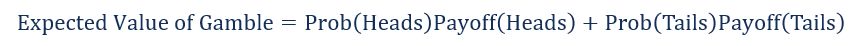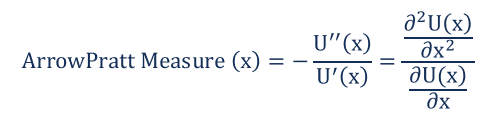# Risk Aversion

The tendency of an economic agent to strictly prefer certainty to uncertainty

## What is Risk Aversion?

Risk aversion refers to the tendency of an economic agent to strictly prefer certainty to uncertainty. An economic agent exhibiting risk aversion is said to be risk averse. Formally, a risk averse agent strictly prefers the expected value of a gamble to the gamble itself.### What is a Gamble?

A gamble consists of three elements:

• A set of outcomes
• The probability associated with each outcome
• The payoff from each outcome

Consider the following example: John and Mark are playing a game. John flips a coin and if it is heads, Mark gives him \$10. If it is tails, Mark gives John \$20.

• A set of outcomes: Heads, Tails
• Probability of Heads = Probability of Tails = ½
• John’s payoff from heads is \$10. John’s payoff from tails is \$20.The general formula for expected value is the following:Therefore, the expected value of the gamble described above is +\$15 for John. He will make \$15 every time he takes part in the gamble. If John is risk averse, then he strictly prefers receiving \$15 with certainty to the gamble.

The risk premium of a gamble is the extra amount required to make an agent indifferent between the gamble and the expected value of the gamble. Conversely, it can also be thought of as the amount of money a risk averse agent will pay to avoid any risk.

In the example above, the expected value of the gamble is \$15. The utility received from the expected value of the gamble is 1.17 (log 15). The expected utility from the gamble is 1.15 (½ log 10 + ½ log 20). It is equal to the utility received when consumption is \$14.

Therefore, the risk premium is \$15 – \$14 = \$1. A risk averse agent is indifferent between a gamble that offers an expected value of \$15 and receiving \$14 with certainty. The consumer would pay up to \$1 to avoid taking the gamble.

### Measures of Risk Aversion

The Arrow-Pratt measure of risk aversion is the most commonly used measure of risk aversion. It analyzes the degree of risk aversion by analyzing the utility representation. The measure is named after two economists: Kenneth Arrow and John Pratt. The Arrow-Pratt formula is given below:Where:

• U’ and U’’ are the first and second derivative of the utility function with respect to consumption x.

### Significance of Risk Aversion

Speaking more practically, risk aversion is an important concept for investors. Investors who are extremely risk-averse prefer investments that offer a guaranteed, or “risk-free”, return. They prefer this even if the return is relatively low compared to higher potential returns that carry a higher degree of risk. For example, extremely risk-averse investors prefer investments such as government bonds and certificates of deposit (CDs) to higher-risk investments such as stocks and commodities.

Investors with a higher risk tolerance – or lower levels of risk aversion – are willing to accept greater levels of risk in exchange for the opportunity to earn higher returns on investment,

Thank you for reading CFI’s explanation of risk aversion. CFI is the official provider of the Financial Modeling and Valuation Analyst (FMVA)™ certification program, designed to transform anyone into a world-class financial analyst.

To keep learning and developing your knowledge of financial analysis, we highly recommend the additional resources below:

• Risk and Return
• Risk Management
• Systematic Risk
• Systemic Risk

### Financial Analyst Training

Get world-class financial training with CFI’s online certified financial analyst training program!

Gain the confidence you need to move up the ladder in a high powered corporate finance career path.

Learn financial modeling and valuation in Excel the easy way, with step-by-step training.